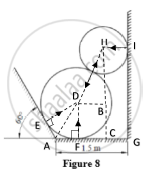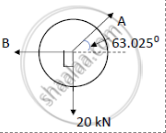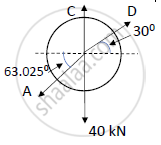Advertisement Remove all ads

# Cylinder a (Diameter 1m, Weight 20 Kn) and Cylinder B (Diameter 1.5m, Weight 40 Kn) Are Arranged as Shown in Figure 8. Find the Reactions at All Contact Points. All Contacts Are Smooth. - Engineering Mechanics

Cylinder A (diameter 1m, weight 20 kN) and cylinder B (diameter 1.5m, weight 40 kN) are arranged as shown in Figure 8. Find the reactions at all contact points. All contacts are smooth.Advertisement Remove all ads

#### SolutionIn ΔDAE and ΔDAF,
DA = DA;
∠DEA = ∠DFE;
By RHS rule , ΔDAE is congruent to ΔDAF
∠DAE = ∠DAF
∠DAE + ∠DAF = 2∠DAE = 180 – 60
∠DAE = ∠DAF = 600
In ΔDAF,

tan (60) (DF)/(AF)

AF = (0.75)/(tan(60) )= 0.433 m

AG = AF + FC + CG
1.5 = 0.433 + DB + HI
1.5 – 0.433 – HI = DB
1.5 – 0.433 – 0.5 = DB
DB = 0.567 m
In ΔDBH,

Cos(D) =(DB)/(DH) = (0.567)/(0.75+.5) = 0.4536

D = cos^(-1) 0.4536 = 63.025^0
Considering cylinder A,Using Lami’s theorem,

20/sin (180-63.025) = A/(sin(90)) = B/(sin(90+63.025))

A = 22.44 kN
B = 10.1795 kN

Considering cylinder B,ΣFx = 0
300 Dcos(30) – A cos(63.025) = 0 …..(1)

D = 11.75 kN

ΣFy = 0

C – 40 + Dsin(30) – Asin(63.025) = 0

C =25.876 kN

The magnitudes of the reaction forces are
A = 22.44 kN
B = 10.1795 kN
C = 25.876 kN
D = 11.75 kN

Concept: Equilibrium of parallel force system
Is there an error in this question or solution?
Advertisement Remove all ads

#### APPEARS IN

Advertisement Remove all ads
Advertisement Remove all ads
Share
Notifications

View all notifications

Forgot password?